This function plots tree tags by status and outputs a list of plots that can be printed on a .pdf file. Each plot shows four subquadrats within a quadrat. The symbols on the plot represent the status of each tree -- not the status of each stem. Although you should likely provide data of only one or two censuses, plot_tag_status_by_subquadrat() will summarize the data to reduce overplotting. The data on the plot summarizes the history of each stem across all censuses provided. Each tag will appear in the plot only once or twice:

• A tag will appear once if it belongs to a tree which status was unique across all censuses provided -- either "alive" or "dead".

• A tag will appear twice if it belongs to a tree which status was "alive" in at least one census, and also "dead" in at least one other census. This feature avoids unintentional overplotting and makes interpreting the plot easier.

plot_tag_status_by_subquadrat(
vft,
x_q = 20,
x_sq = 5,
y_q = 20,
y_sq = 5,
bl = 1,
br = 2,
tr = 3,
tl = 4,
show_page = TRUE,
point_shape = c(19, 4),
point_size = 1.5,
tag_size = 3,
theme = theme_tag_status(),
move_edge = 0
)

## Arguments

vft

A ForestGEO ViewFullTable (dataframe).

x_q, y_q

Size in meters of a quadrat's side. For ForestGEO sites, a common value is 20.

x_sq, y_sq

Size in meters of a subquadrat's side. For ForestGEO-CTFS sites, a common value is 5.

NULL or -1. NULL defines the first column of subquadrats as 1. -1 defines the first column of subquadrats as 0.

subquad_offset = NULL    subquad_offset = -1
---------------------    -------------------
14 24 34 44             04 14 24 34
13 23 33 43             03 13 23 33
12 22 32 42             02 12 22 32
11 21 31 41             01 11 21 31


bl, br, tr, tl

Number or character giving the label of the four subquadrats on each or the four divisions of a quadrat: bottom left (bl), bottom right (br), top right (tr), and top left (tl).

A string to use as a title.

show_page

Logical; FALSE removes the page label from the plot title.

Logical; FALSE removes subquadrat labels on each plot.

point_shape

A vector of two numbers giving the shape of the points to plot (see possible shapes in the documentation of ?graphics::points(), under the section entitled 'pch' values).

point_size

A number giving points size. Passed to ggplot2::geom_point().

tag_size

A number giving tag size. Passed to ggrepel::geom_text_repel.

theme

An object of class "theme".

move_edge

A number to adjust the extension of the grid lines beyond the plot limits.

## Value

A list of objects of class "ggplot".

## Acknowledgment

Useful ideas and guidance came from Suzanne Lao, Stuart Davis, Shameema Jafferjee Esufali, David Kenfack and Anudeep Singh. Anudeep Sinh also wrote the algorithm to calculate subquadrats.

graphics::points(), ggplot2::geom_point(), ggplot2::theme() header_tag_status(), theme_tag_status(), fgeo.tool::add_subquad(), ggrepel::geom_text_repel.

Other plot functions: autoplot.fgeo_habitat(), autoplot.sp_elev(), autoplot_by_species.sp_elev(), elev(), plot_dbh_bubbles_by_quadrat(), sp_elev(), sp()

Other functions to list plots from ForestGEO ViewFullTable: plot_dbh_bubbles_by_quadrat()

Other functions to plot tag status: header_tag_status(), theme_tag_status()

## Examples

assert_is_installed("fgeo.x")

# Create a small VieFullTable
first <- function(x) x %in% sort(unique(x))

p[1:2]
#> $621_1 #> Warning: Use of prep_df$tag is discouraged. Use tag instead.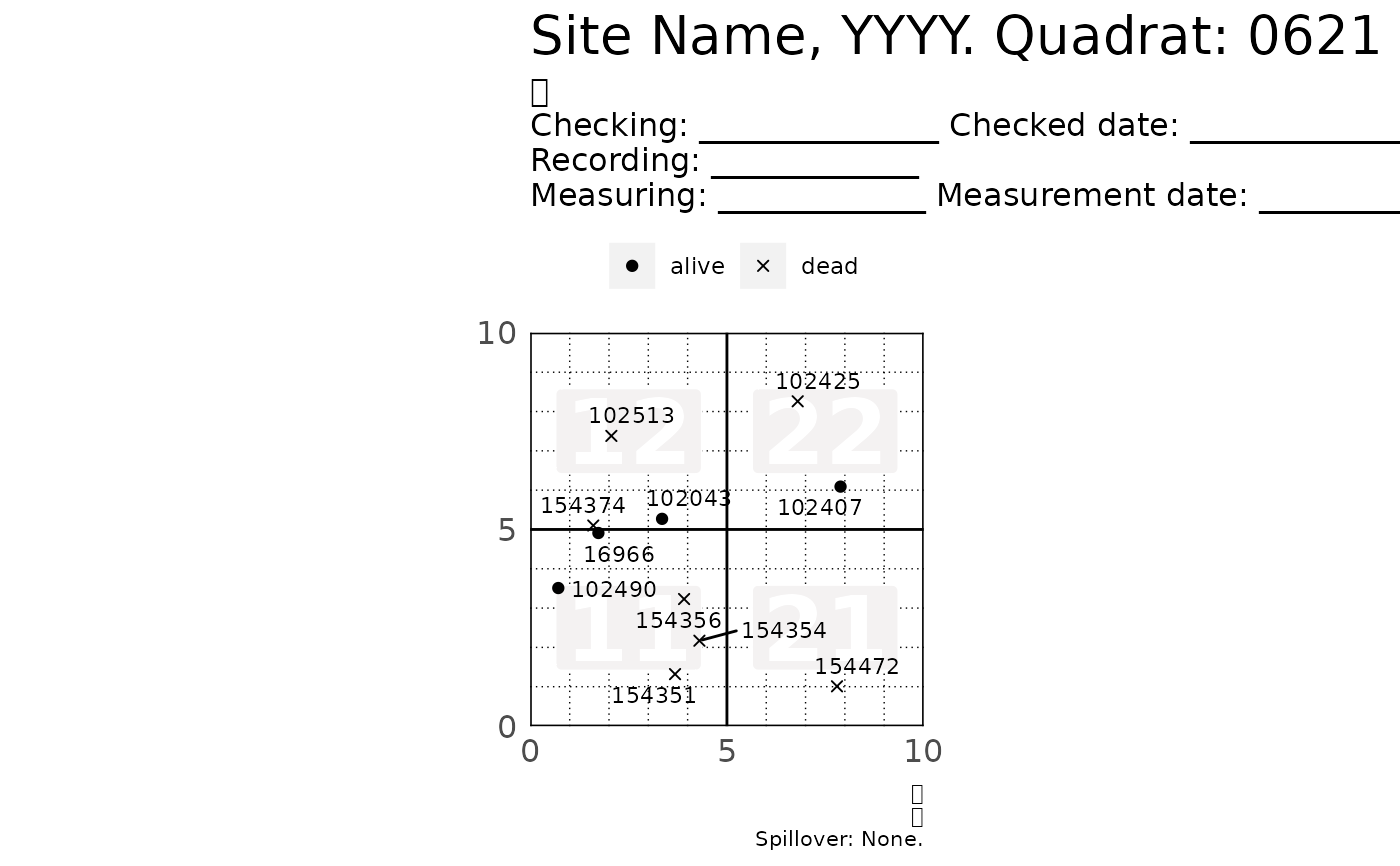#>
#> $621_2 #> Warning: Use of prep_df$tag is discouraged. Use tag instead.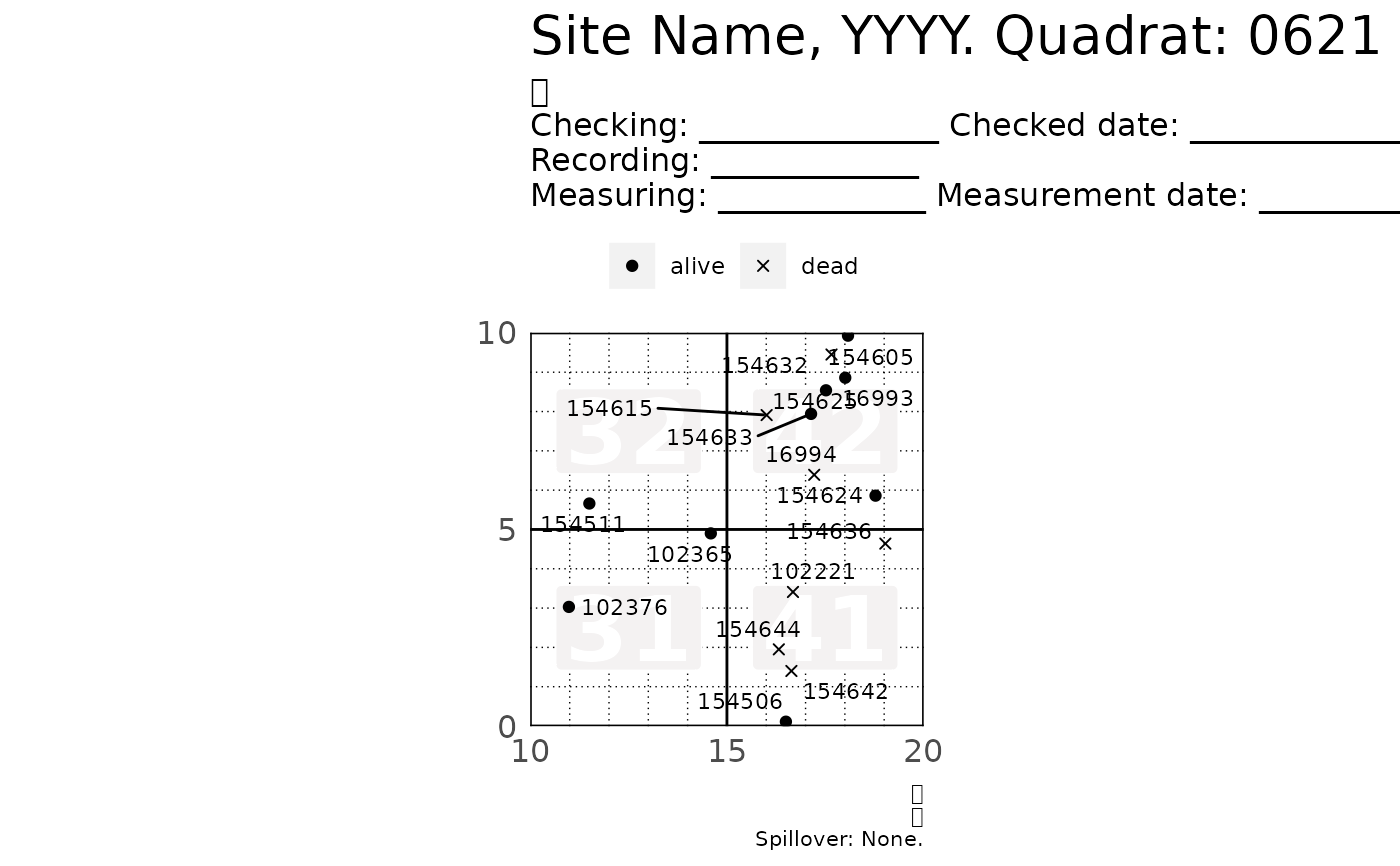#>

# To print all plots into a .pdf file see ?pdf()
#> $621_1 #> Warning: Use of prep_df$tag is discouraged. Use tag instead.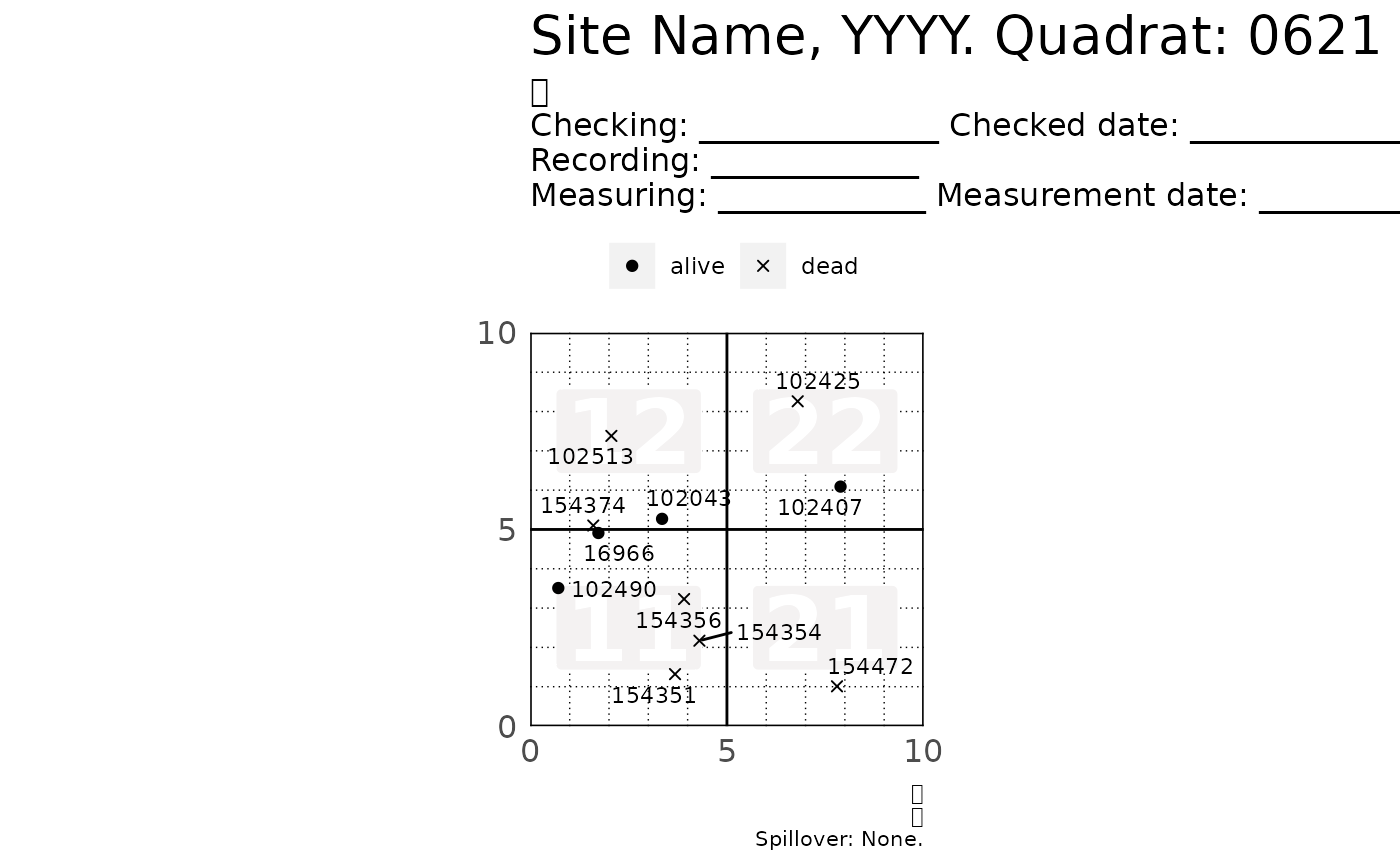#>
#> $621_2 #> Warning: Use of prep_df$tag is discouraged. Use tag instead.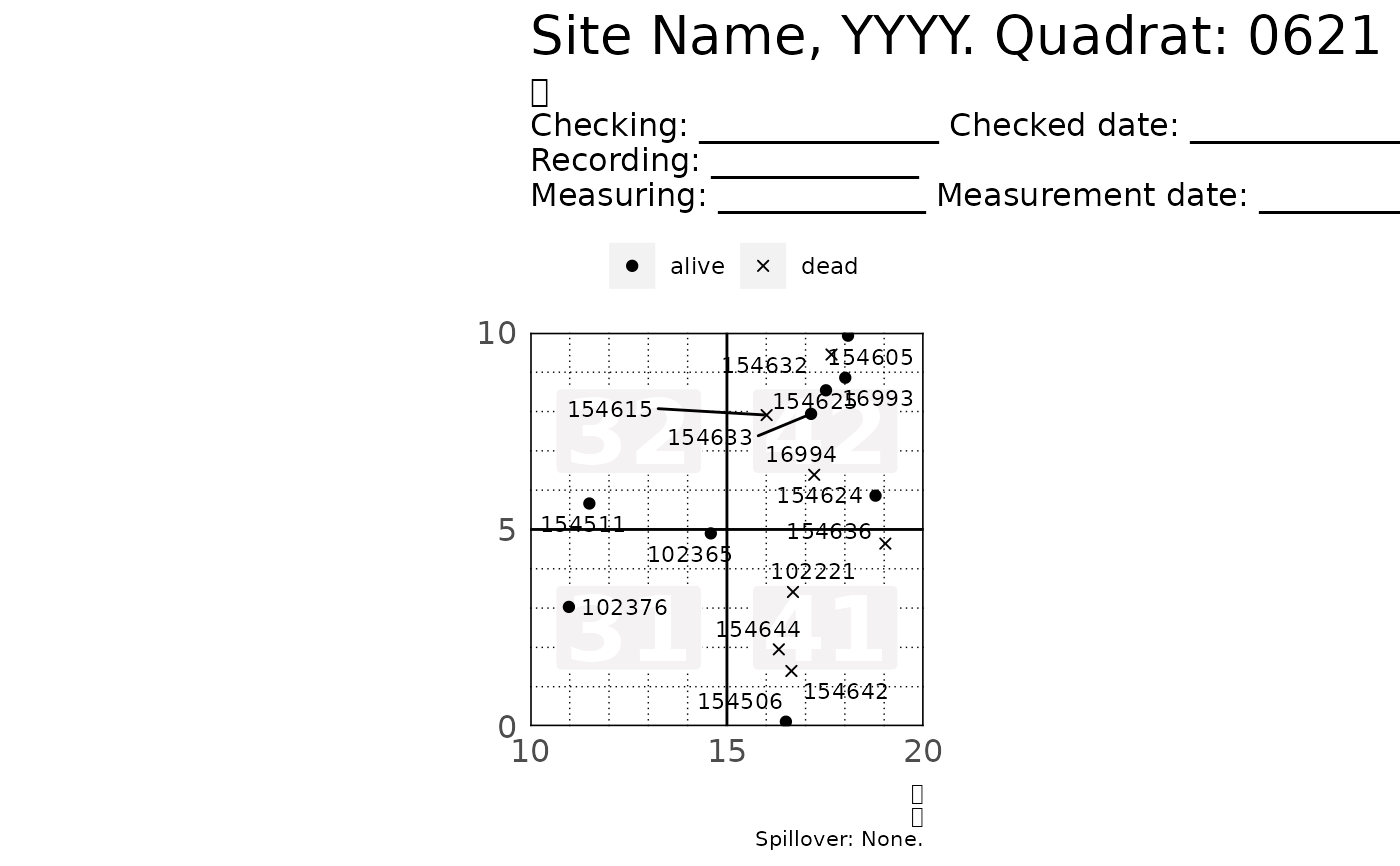#>
#> $621_3 #> Warning: Use of prep_df$tag is discouraged. Use tag instead.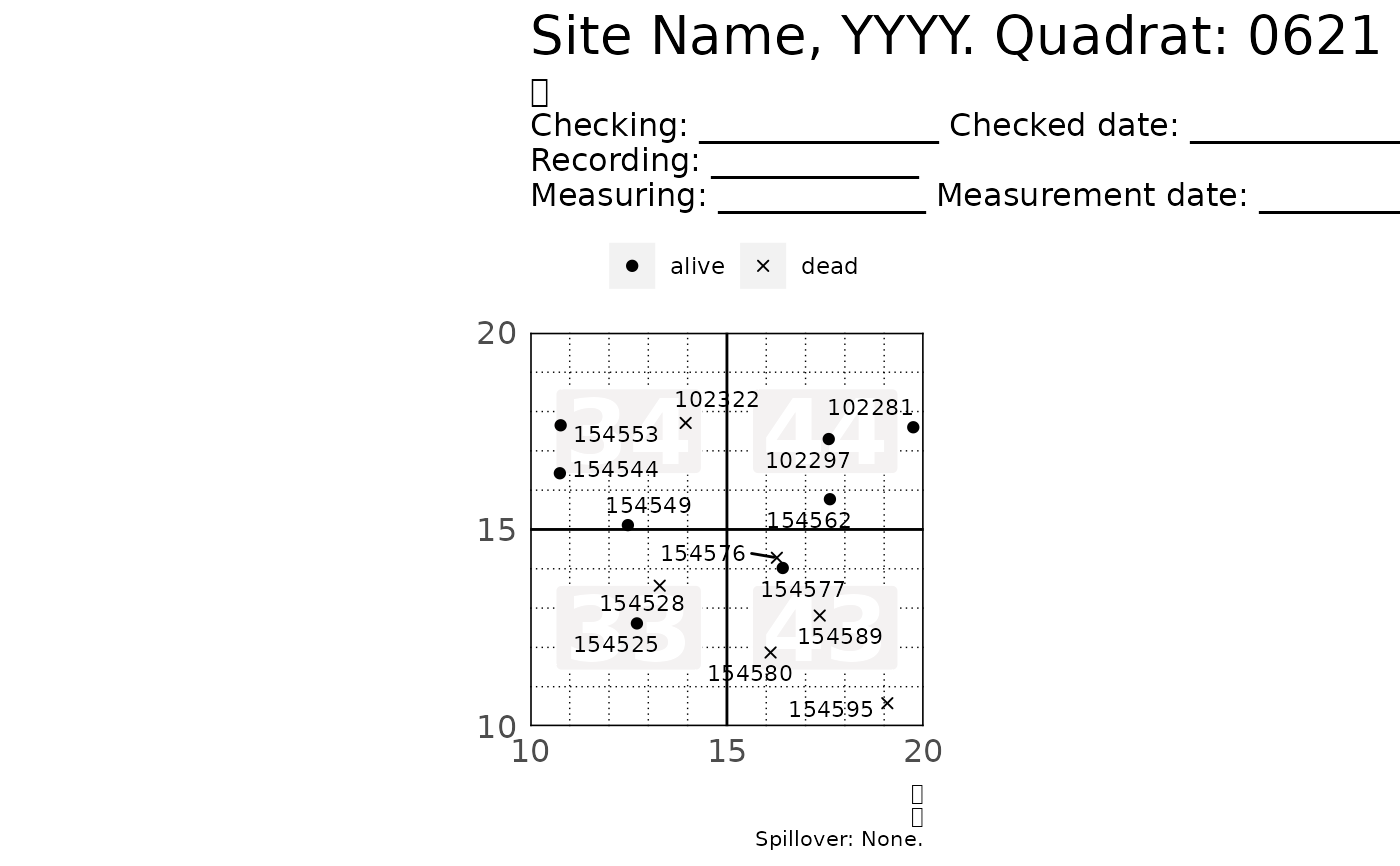#>
#> $621_4 #> Warning: Use of prep_df$tag is discouraged. Use tag instead.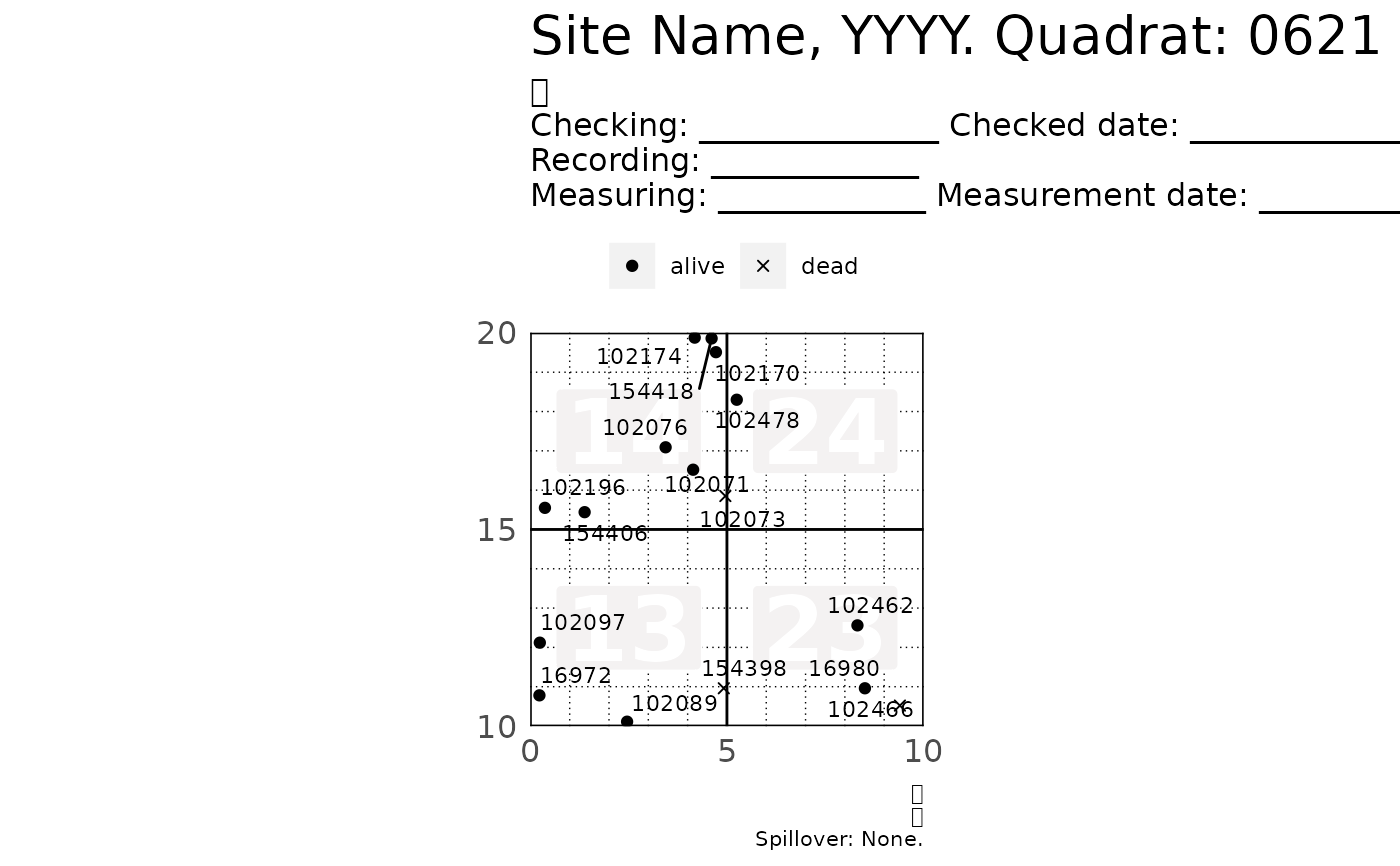#>

# Be careful if filtering by DBH: You may unintentionally remove dead trees.
# * If you filter by DBH, you loose the dead trees becaue their DBH = NA
# * You should explicietly inlcude missing DBH values with is.na(DBH)
include_missing_dbh <- subset(small_vft, DBH > 20 | is.na(DBH))
# Showing only the first plot to keep the output short
p[]
#> Warning: Use of prep_df$tag is discouraged. Use tag instead.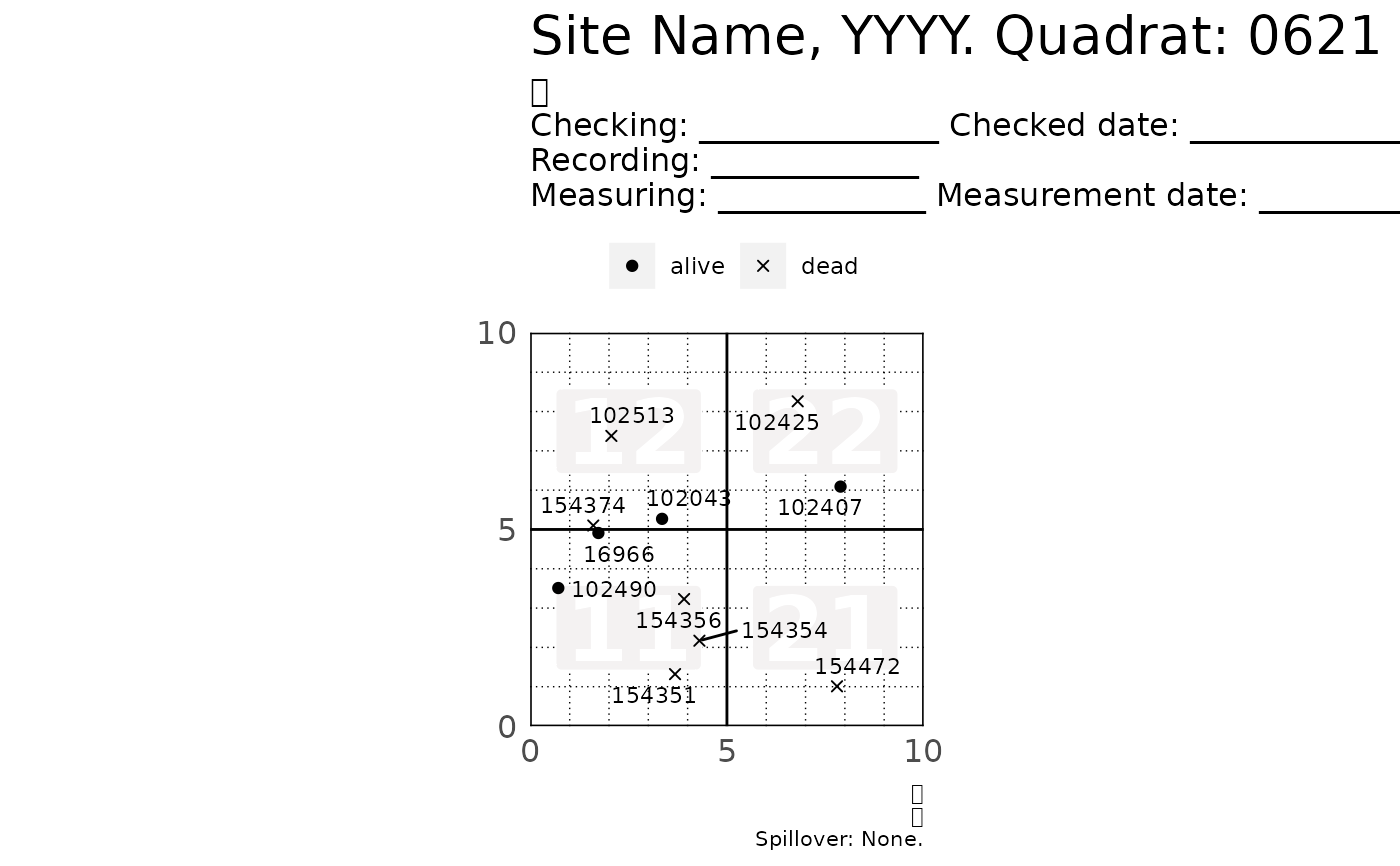# Customizing the maps ---------------------------------------------------- # Common tweaks p <- plot_tag_status_by_subquadrat( small_vft, title_quad = "BCI 2012. Quadrat: ", bl = "bottom-left", br = "bottom-right", tr = "top-right", tl = "top-left", header = "Line 1: _________\nLine 2:\nLine 3:.....................", subquad_offset = -1, point_size = 3, point_shape = c(17, 6), tag_size = 2, move_edge = 0.5 ) p[] #> Warning: Use of prep_df$tag is discouraged. Use tag instead.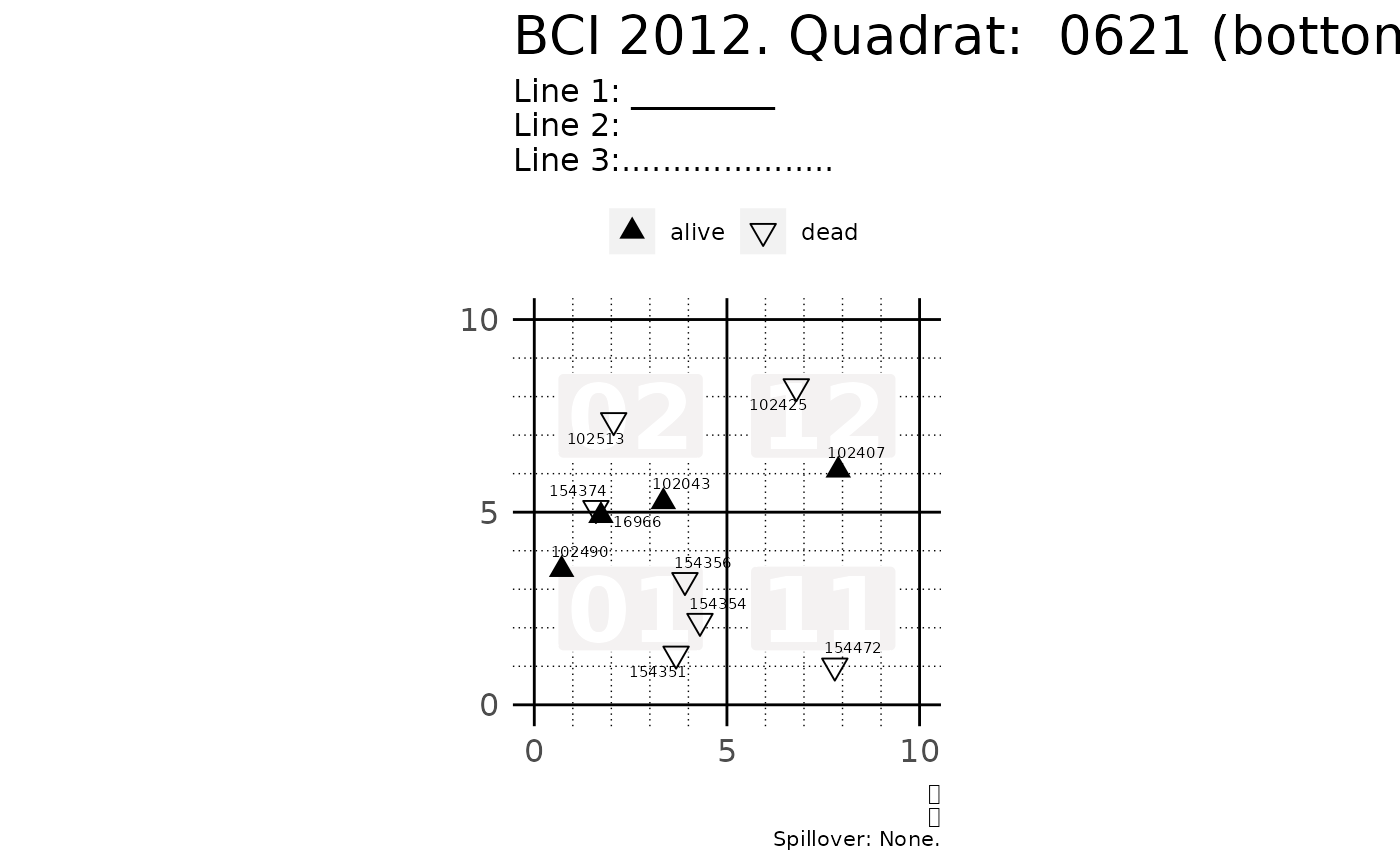# Skip R CMD check for speed
# \donttest{
small_vft,
show_page = FALSE,
)
p[]
#> Warning: Use of prep_df$tag is discouraged. Use tag instead.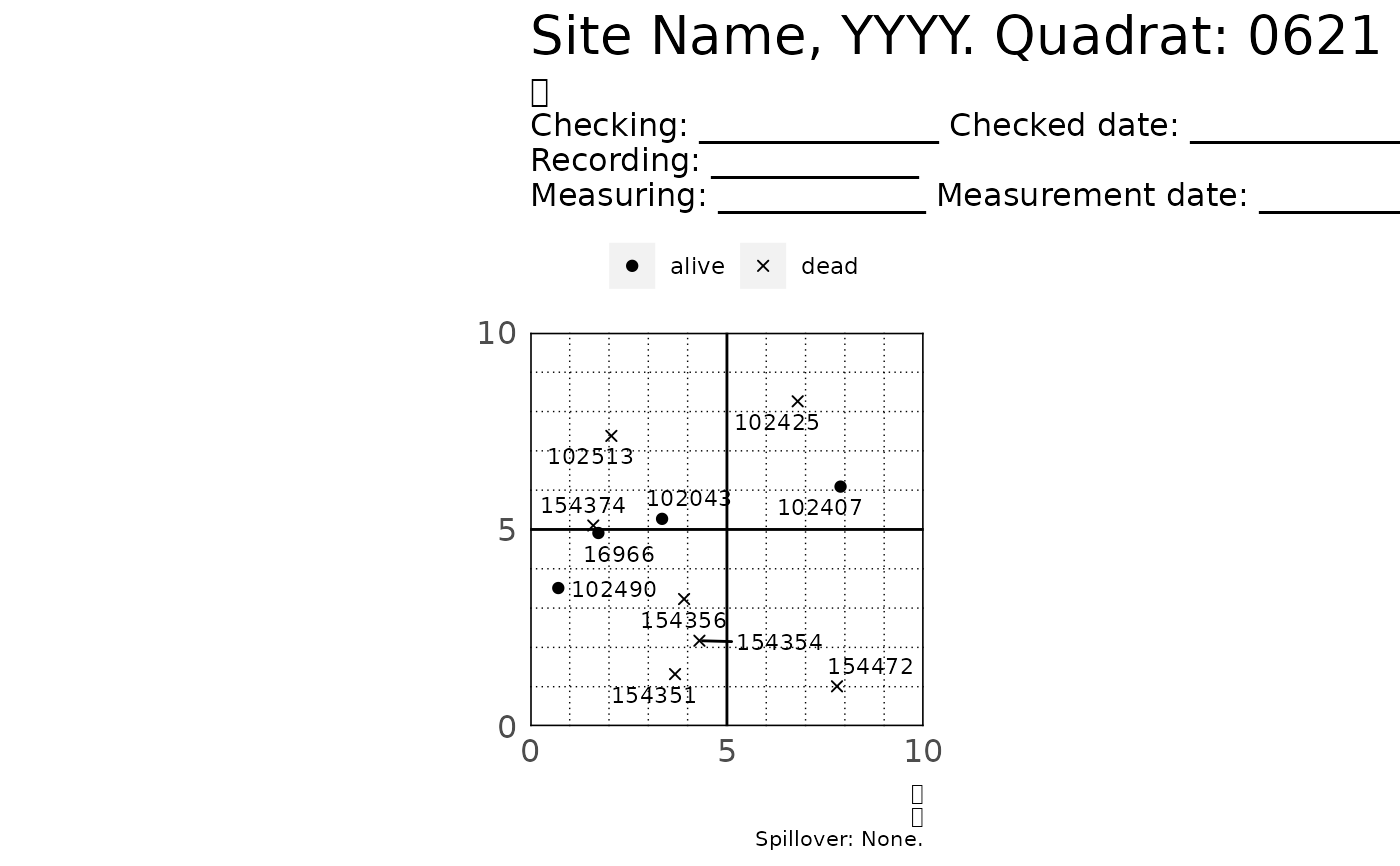# Themes library(ggplot2) p <- plot_tag_status_by_subquadrat(small_vft, theme = theme_gray()) p[] #> Warning: Use of prep_df$tag is discouraged. Use tag instead.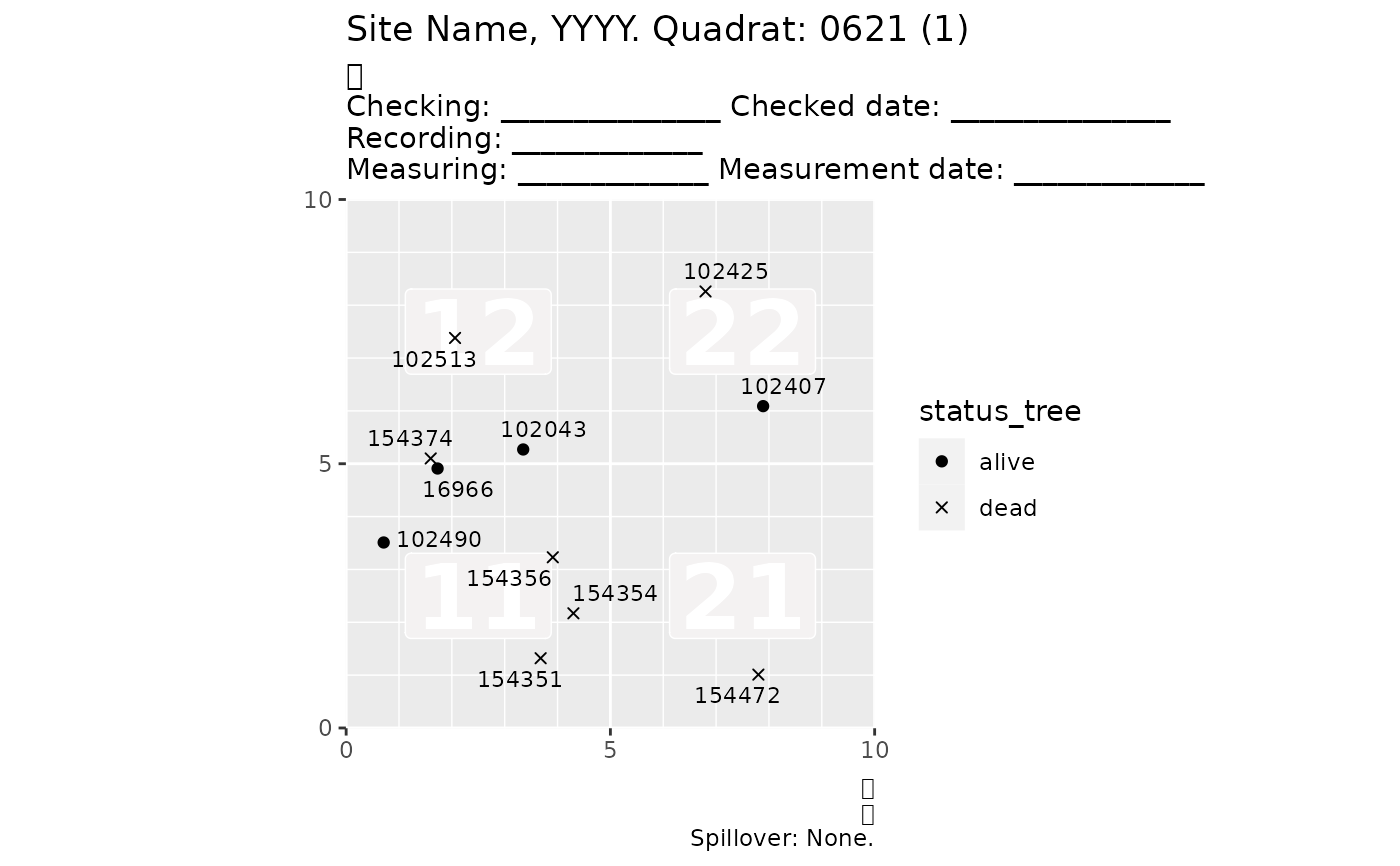# Tweaking the default theme of plot_tag_status_by_subquadrat()
# For many more options see ?ggplot2::theme
small_tweak <- theme_tag_status(legend.position = "bottom")
p <- plot_tag_status_by_subquadrat(small_vft, theme = small_tweak)
p[]
#> Warning: Use of prep_df\$tag is discouraged. Use tag instead.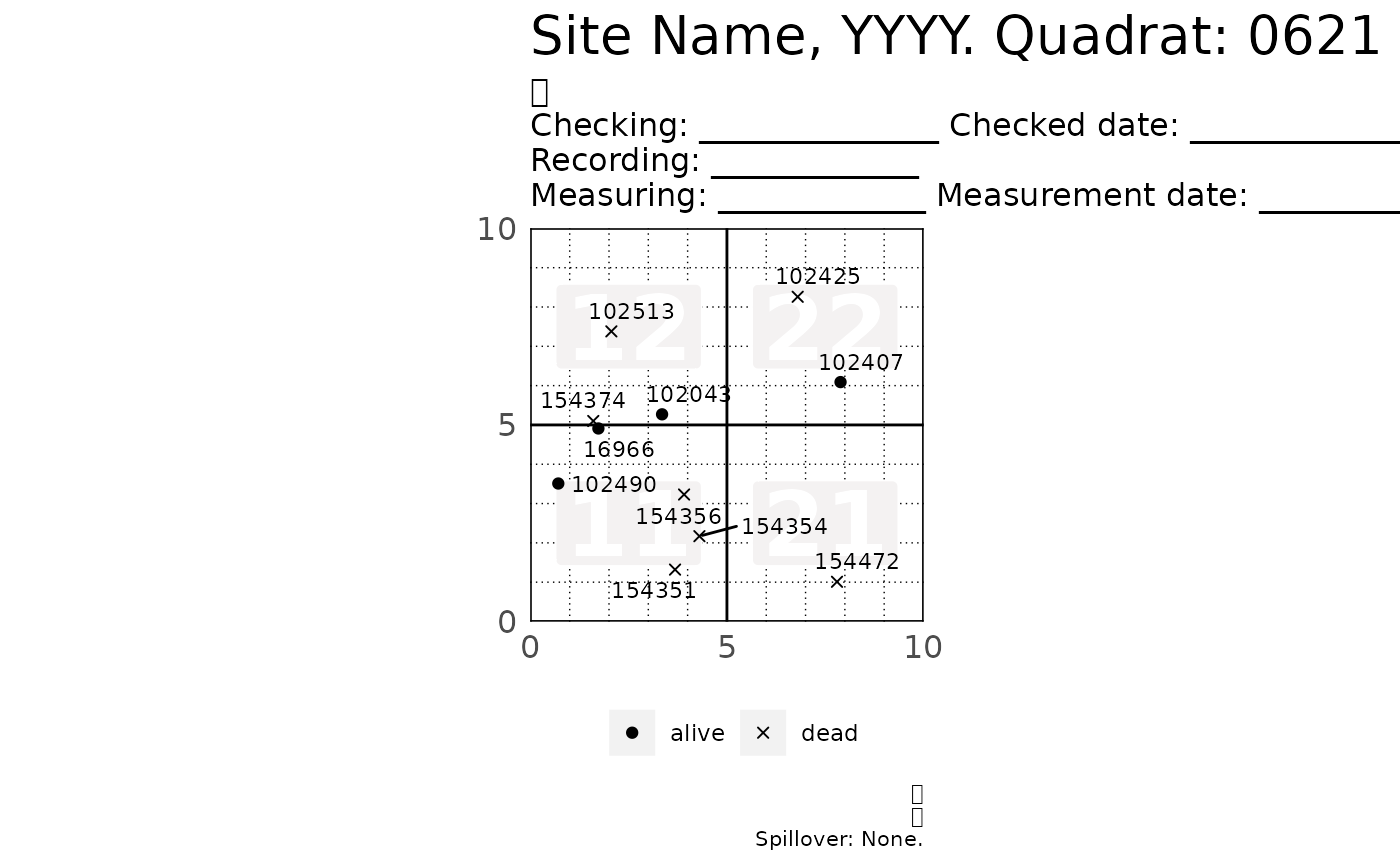# }